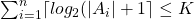# Microcode Bit Optimization

The Problem: Microcode Bit Optimization.  This is problem PO10 in the appendix

The description: We have a set A of “microcommands”.  We take groups of these commands to create a set C of “microinstructions”.   We would like to partition our set A of commands into groups where each command uses at most one instruction from each group. Give an integer K, can we make such a split of A (into groups A1..An) such that?

(I’ll note here that the definition in G&J requires this split into groups to be a partition into disjoint sets, while the paper we will be using allows the split to be a covering where a microcommand can appear in multiple Ai)

Example: The log part is there because we can represent groups as binary numbers.  The idea is that if we only have one group, we can represent microinstructions as a binary string of which microcommands we are using.  For example, if we make every microcommand its own group, then a microinstruction can be represented in binary: 101 might mean microcommand #5.  This makes our microinstructions smaller, but each microinstruction can only represent one microcommand.

In the other extreme, we can represent a microinstruction as a binary string of what microcommands are being used. In this model. the instruction “10101” says that we are using the first, third, and fifth microcommand, but not any of the others.  This lets us have longer microinstructions that use several microcommands at once.

So the problem is asking: How many groups are needed to minimize the representation of the microinstructions and mirocommands in a given program?  So, for example, suppose we had 8 microcommands and the following microinstructions (written as a binary string of what microcommands are used):

• 1000 1111
• 0100 0011
• 0010 1100
• 0001 0101

Notice that our 4 microinstructions never have 2 microcommands used from the 4 leftmost commands at the same time, so we can represent that chunk of the instruction as 2 bits (counting from 0-3, representing what command we are using).  We will need 4 bits to represent the rightmost 4 microcommands because all of those microcommands are in use in the first microinstruction.  But if we change the last 4 bits of the first instruction to 1011, then no instruction uses both the second and third commands out of those 4 bits, and we could represent those 2 commands using 1 bit (maybe a 0 means we use the second microcommand, and a 1 means we use the third one)

Reduction: G&J say to use 3DM, but the paper I found by Robertson uses CNF-SAT.  We assume that no clause has both a literal and its negation (because if it did the clause is automatically true), and also we never have a literal appear both with a variable and its negation in different clauses (we can add extra variables to remove this situation).

Suppose our formula has clauses T1..Tp and variables x1..xk.  Each of these will be its own microcommand.

The microinstructions will be:

• An instruction {xi, xj} for all i < j.
• An instruction {xi, Tj} if xi (or its negation) is not in clause Tj
• An instruction {Ti, Tj} if those 2 clauses do not share any literals (they might share variables)

Define a maximal compatibility class (MCC) as a set of mutually compatible instructions that cannot add any other instruction without breaking compatibility.  Our job is to make these classes (the bigger the classes, the more items we can put in each subset)

We can make an MCC Ri out of xi and all of the Tj‘s that have xi in the clause.  We can make a second MCC Si out of xi and all of the Tj‘s that have ~xi in the clause.  Our goal is to use just k of these classes (one for each variable) to cover all of the instructions.

So, suppose the formula is satisfiable.  Then for all variables that are set to true in the satisfying arrangement, we use the Ri class of the variable, and for all variables that are set to false, we use the Si class for that variable.  Since we have chosen either Ri or Sfor each variable, each xi is covered.  Since the formula is satisfiable, all Ti are also covered by whatever variable makes the clause true.

In the other direction, if we have exactly k R’s and S’s and cover all of the T instructions, then the choice of R or S for each variable will give a consistent way to satisfy each clause.

We are not quite done, because of the requirement to use the log formula to measure the size of the sets, instead of just minimizing the number of the sets.  The paper goes into the algebraic details of manipulating these sets to satisfy those requirements.

Difficulty: 8. The log formula stuff is almost longer than the rest of the proof, which is annoying.  Other than that, the reduction is relatively straightforward, I think.